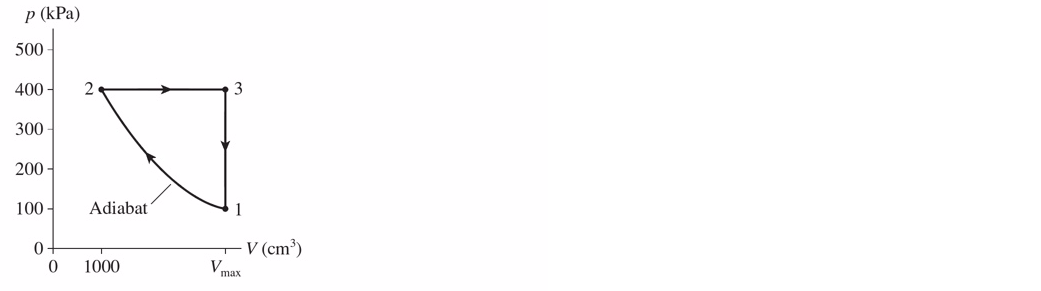# Problem: The engine shown in the following figure uses 0.1 mol of a monoatomic ideal gas. What is the thermal efficiency of the engine if Vmax = 1741 cm3?

###### FREE Expert Solution
88% (194 ratings)
###### Problem Details

The engine shown in the following figure uses 0.1 mol of a monoatomic ideal gas. What is the thermal efficiency of the engine if Vmax = 1741 cm3?Frequently Asked Questions

What scientific concept do you need to know in order to solve this problem?

Our tutors have indicated that to solve this problem you will need to apply the Heat Engines & PV Diagrams concept. You can view video lessons to learn Heat Engines & PV Diagrams. Or if you need more Heat Engines & PV Diagrams practice, you can also practice Heat Engines & PV Diagrams practice problems.